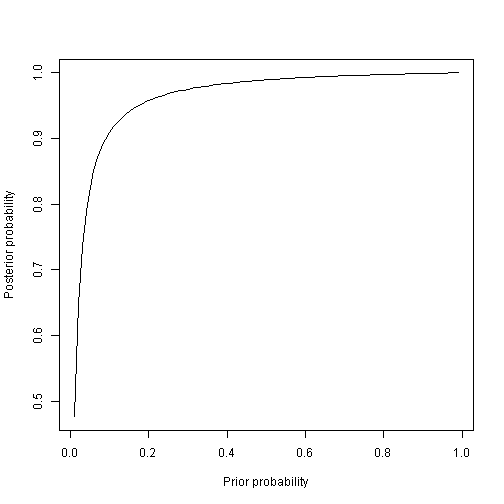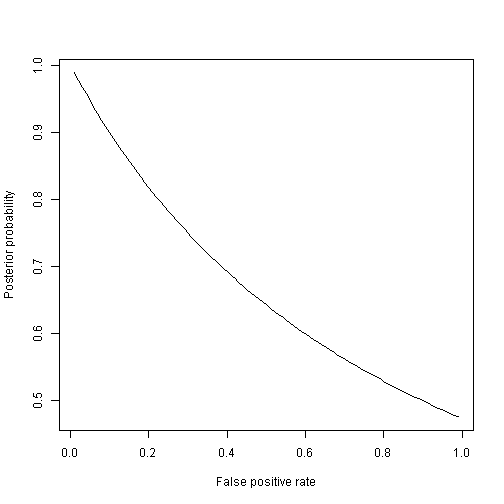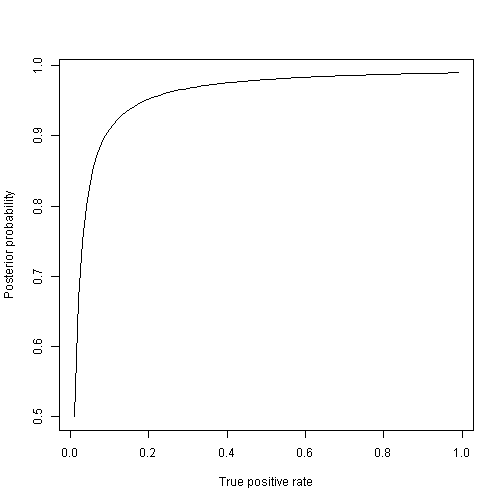# HIV testing example of Bayes' rule

In this example, the patient takes an HIV test and we compute the posterior probability that the patient has HIV. Let

$$p = \mbox{prior probability that the patient has HIV}$$

$$q_0 = \mbox{probability of a positive test given the patient is negative}$$

$$q_1 = \mbox{probability of a positive test given the patient is positive}$$

## Compute the posterior probability the patient has HIV given a positive test

post_prob<-function(p,q0,q1){
p*q1/(p*q1+(1-p)*q0)
}


## Base case

p  <- 0.50   # Prior probability
q0 <- 0.01   # False positive probability
q1 <- 0.90   # True positive probability

post_prob(p,q0,q1)

##  0.989011


## Effect of the prior

grid  <- seq(0.01,0.99,.01)

plot(grid,post_prob1(grid,q0,q1),
type="l",
xlab="Prior probability",
ylab="Posterior probability")## Effect of the likelihood - false positive rate

plot(grid,post_prob1(p,grid,q1),
type="l",
xlab="False positive rate",
ylab="Posterior probability")## Effect of the likelihood - true positive rate

plot(grid,post_prob1(p,q0,grid),
type="l",
xlab="True positive rate",
ylab="Posterior probability")## Monte Carlo approximation:

An alternative to the exact posterior calculation is a Monte Carlo approximation. Here we will generate many samples from the joint distribution of $$\theta$$ and $$Y$$ to approximate their joint distribution, and then use these samples to estimate the posterior.

n     <- 10000
theta <- NULL
Y     <- NULL

#start sampling
for(i in 1:n){
theta[i] <- rbinom(1,1,p)
prob     <- ifelse(theta[i]==1,q1,q0)
Y[i]     <- rbinom(1,1,prob)
}

table(Y,theta)/n  # Approximate joint distribution

##    theta
## Y        0      1
##   0 0.4989 0.0467
##   1 0.0054 0.4490

mean(theta[Y==1]) # Approximate conditional probability

##  0.9881162